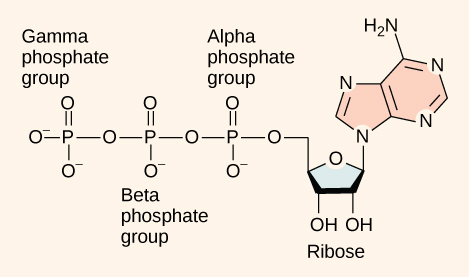The MCAT CARS Strategy Course begins January 28!

MCAT Content / Principles Of Bioenergetics / Phosphoryl Group Transfers And Atp 1d

### Phosphoryl Group Transfers and ATP

Topic: Principles Of Bioenergetics

Adenine triphosphate (ATP) is used to power the majority of energy-requiring cellular reactions as it has an adenosine backbone with three phosphate groups attached.Adenosine triphosphate (ATP) is comprised of the molecule adenosine bound to three phosphate groups. Adenosine is a nucleoside consisting of the nitrogenous base adenine and the five-carbon sugar ribose. The three phosphate groups, in order of closest to furthest from the ribose sugar, are labeled alpha, beta, and gamma. Together, these chemical groups constitute an energy powerhouse. The two bonds between the phosphates are equal high-energy bonds (phosphoanhydride bonds) that, when broken, release sufficient energy to power a variety of cellular reactions and processes.

The bond between the beta and gamma phosphate is considered “high-energy” because when the bond breaks, the products [adenosine diphosphate (ADP) and one inorganic phosphate group (Pi)] have a lower free energy than the reactants (ATP and a water molecule). ATP breakdown into ADP and Pi is called hydrolysis because it consumes a water molecule (hydro-, meaning “water”, and lysis, meaning “separation”). This is an example of an exergonic (exothermic) reaction which releases heat energy to the surroundings.

ATP is hydrolyzed into ADP in the following reaction:

Like most chemical reactions, the hydrolysis of ATP to ADP is reversible. The reverse reaction combines ADP + Pi to regenerate ATP from ADP. Since ATP hydrolysis releases energy, ATP synthesis must require an input of free energy.

ADP is combined with a phosphate to form ATP in the following reaction:

Exactly how much free energy (∆G) is released with the hydrolysis of ATP, and how is that free energy used to do cellular work? The calculated ∆G for the hydrolysis of one mole of ATP into ADP and Pi is −7.3 kcal/mole (−30.5 kJ/mol). However, this is only true under standard conditions, and the ∆G for the hydrolysis of one mole of ATP in a living cell is almost double the value at standard conditions: 14 kcal/mol (−57 kJ/mol). Free energy is a measure of the energy released from a reaction that can be used to do work.

ATP is a highly unstable molecule. Unless quickly used to perform work, ATP spontaneously dissociates into ADP + Pi, and the free energy released during this process is lost as heat. To harness the energy within the bonds of ATP, cells use a strategy called energy coupling.

One example of energy coupling using ATP involves a transmembrane ion pump that is extremely important for cellular function. This sodium-potassium pump (Na+/K+pump) drives sodium out of the cell and potassium into the cell. A large percentage of a cell’s ATP is spent powering this pump because cellular processes bring a great deal of sodium into the cell and potassium out of the cell. The pump constantly works to stabilize cellular concentrations of sodium and potassium. In order for the pump to turn one cycle (exporting three Na+ ions and importing two K+ ions), one molecule of ATP must be hydrolyzed. The energy released from the hydrolysis of ATP is coupled with the energy required to power the pump and transport Na+ and K+ ions. ATP performs cellular work using this basic form of energy coupling through phosphorylation.ATP group transfers involve the covalent transfer of a portion of the ATP molecule to a substrate (e.g. an enzyme active site), which in turn makes subsequent metabolic reactions involving this substrate more thermodynamically favorable. The substrates are commonly coupled to biological reactions that have a large positive standard free energy change.

Practice Questions

Basic concepts in bioenergetics: phosphoryl group transfers and ATP hydrolysis

The thermodynamics of ATP hydrolysis in living cells

MCAT Official Prep (AAMC)

Practice Exam 1 C/P Section Passage 6 Question 32

Practice Exam 2 C/P Section Passage 4 Question 20

Practice Exam 1 B/B Section Passage 5 Question 25

Key Points

• Adenosine triphosphate is composed of the nitrogenous base adenine, the five-carbon sugar ribose, and three phosphate groups.

• ATP is hydrolyzed to ADP in the reaction ATP+H2O→ADP+Pi+ free energy; the calculated ∆G for the hydrolysis of 1 mole of ATP is -57 kJ/mol.

• ADP is combined with a phosphate to form ATP in the reaction ADP+Pi+free energy→ATP+H2O.

• The energy released from the hydrolysis of ATP into ADP is used to perform cellular work, usually by coupling the exergonic reaction of ATP hydrolysis with endergonic reactions.

• Sodium-potassium pumps use the energy derived from exergonic ATP hydrolysis to pump sodium and potassium ions across the cell membrane while phosphorylation drives the endergonic reaction.

• ATP group transfers involve the covalent transfer of a portion of the ATP molecule to a substrate (e.g. an enzyme active site), which in turn makes subsequent metabolic reactions involving this substrate more thermodynamically favorable.

Key Terms

energy coupling: energy coupling occurs when the energy produced by one reaction or system is used to drive another reaction or system

endergonic: describing a reaction that absorbs (heat) energy from its environment

exergonic: describing a reaction that releases energy (heat) into its environment

free energy: gibbs free energy (∆G) is a thermodynamic potential that measures the useful or process-initiating work obtainable from a thermodynamic system at a constant temperature and pressure

hydrolysis: a chemical process of decomposition involving the splitting of a bond by the addition of water

adenosine triphosphate (ATP): the primary energy unit used in cells